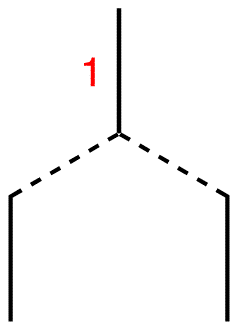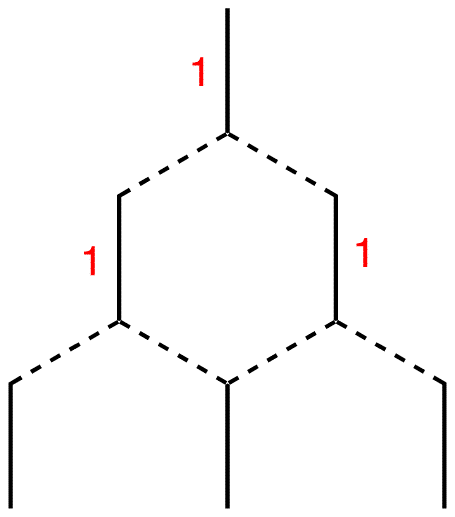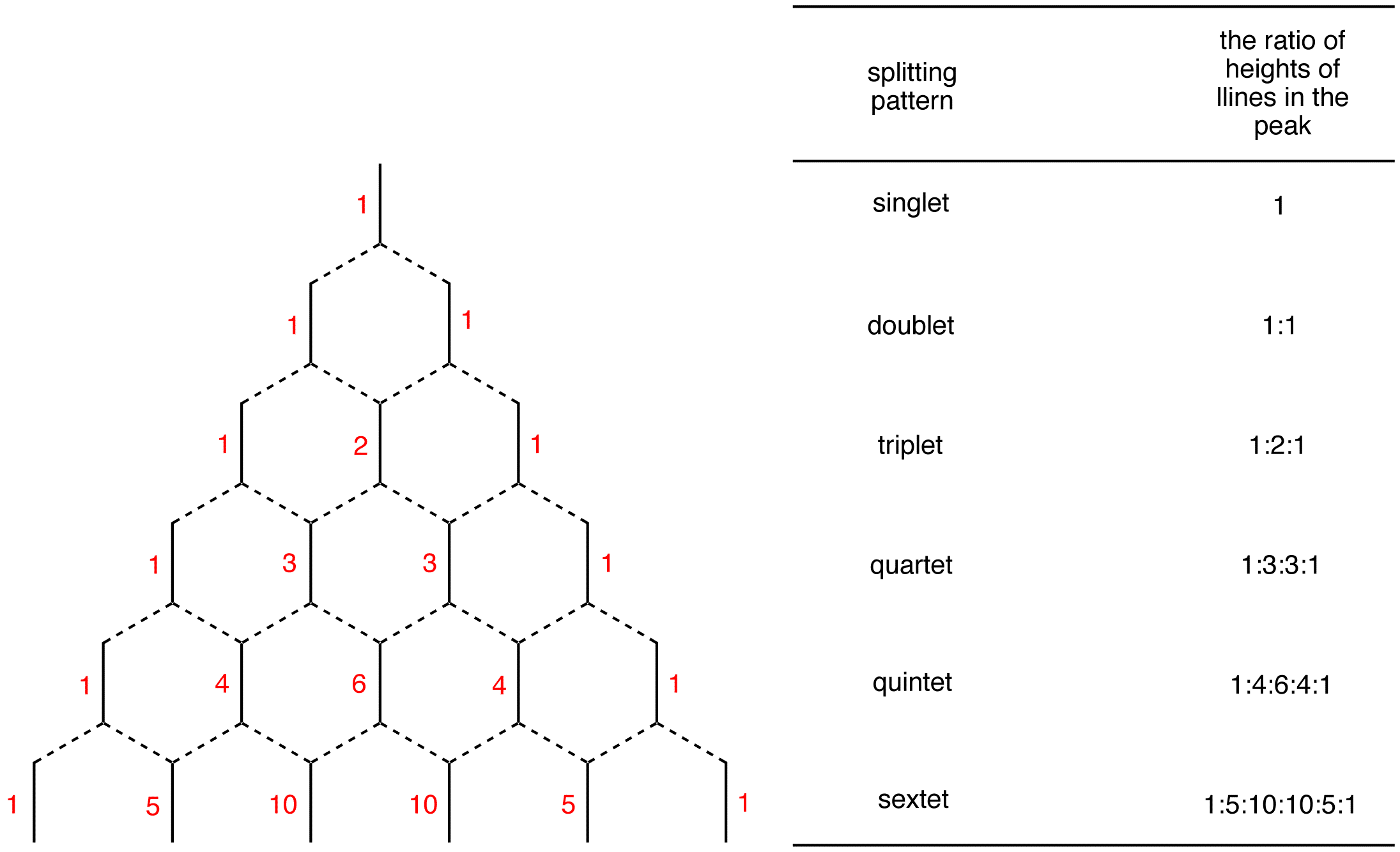Skip to main content

# Pascal’s Triangle Construction

The Pascal’s triangle is a graphical device used to predict the ratio of heights of lines in a split NMR peak. To construct the Pascal’s triangle, use the following procedure.

Step 1: Draw a short, vertical line and write number one next to it.Step 2: Draw two vertical lines underneath it symmetrically.Step 3: Connect each of them to the line above using broken lines.Step 4: Each of the two lines is connected to the single line above, which carries the number one. Therefore, write number one next to each line.Step 5: Draw three vertical lines symmetrically underneath the two lines.Step 6: Connect each of them to the nearest line(s) above.Step 7: Each terminal line is connected to one line above, which carries the number one. Therefore, write number one next to each of them. The internal line is connected to two lines above, each carrying the number one. 1 + 1 = 2; write number two next to the internal line.Continue the process as far down as necessary.